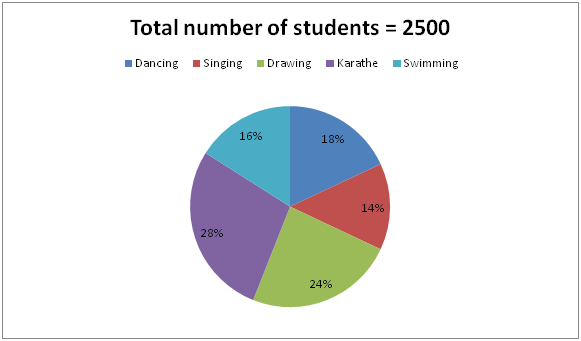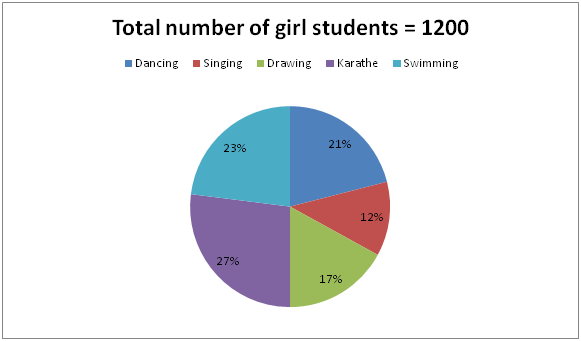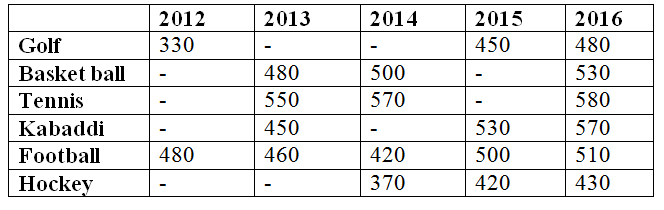# IBPS Clerk Mains Quantitative Aptitude Questions 2019 – (Day-7)

Dear Aspirants, Our IBPS Guide team is providing new pattern Quantitative Aptitude Questions for IBPS Clerk Mains 2019 so the aspirants can practice it on a daily basis. These questions are framed by our skilled experts after understanding your needs thoroughly. Aspirants can practice these new series questions daily to familiarize with the exact exam pattern and make your preparation effective.

Check here for IBPS Clerk Prelims Mock Test 2019

[WpProQuiz 7440]

Data Interpretation

Directions (Q. 1 – 5): Study the following information carefully and answer the given questions?

The following pie chart – 1 shows the percentage distribution of total number of students (Boys + Girls) enrolled for different activities in School A and the pie chart -2 shows the percentage distribution of total number of girl students enrolled for different activities in School A.1) Total number of boy students enrolled for Dancing and Singing together is approximately what percentage of total number of girl students enrolled for same activities?

a) 86 %

b) 124 %

c) 102 %

d) 68 %

e) 74 %

2) Find the difference between the total number of students enrolled for Drawing and Karathe together to that of total number of girl students enrolled for Karathe and Swimming together?

a) 560

b) 700

c) 850

d) 920

e) None of these

3) Find the ratio between the total number of boy students enrolled for Dancing and Swimming together to that of total number of girl students enrolled for Drawing and Karathe together?

a) 143 : 212

b) 125 : 198

c) 133 : 248

d) 161 : 264

e) None of these

4) Find the average number of boy students enrolled for Singing, Drawing and Karathe together?

a) 326

b) 378

c) 412

d) 434

e) None of these

5) Total number of students enrolled for Singing and Swimming together is approximately what percentage more/less than the total number of girl students enrolled for Dancing, Drawing and Karathe together?

a) 16 % less

b) 4 % more

c) 4 % less

d) 25 % less

e) 16 % more

Data Interpretation

Directions (Q. 6 – 10): Study the following information carefully and answer the given questions?

The following table shows the total amount spent (In crores) for 5 different sports in 6 different years in India. Some values are missing.6) If the ratio between the total amount spent on Golf to that of basket ball in the year 2012 is 3 : 4 and the average amount spent on Basket ball in all the given years together is 488 crore, then find the total amount spent on Basket ball in the year 2015?

a) 460 Crore

b) 490 Crore

c) 510 Crore

d) 540 Crore

e) None of these

7) If the total amount spent on golf in the year 2012 and 2013 together is 750 crore and the total amount spent on Hockey in the year 2013 is 80 crore less than the total amount spent on Hockey in the year 2015, then find the average amount spent in the year 2013 for all given sports together?

a) 420 crore

b) 380 crore

c) 400 crore

d) 450 crore

e) None of these

8) The total amount spent on Basket ball and Kabaddi in the year 2013 is approximately what percentage of total amount spent on Hockey in the year 2015 and 2016 together?

a) 95 %

b) 110 %

c) 130 %

d) 75 %

e) 120 %

9) Find the difference between the total amount spent on Football to that of Hockey in all the given years together, if the ratio between the total amount spent on Hockey in the year 2012 and 2013 is 16 : 17 and the total amount spent on Hockey in the year 2013 is 340 Crore?

a) 490 crore

b) 450 crore

c) 510 crore

d) 530 crore

e) None of these

10) The total amount spent on Kabaddi in the year 2015 and 2016 together is approximately what percentage more/less than the total amount spent on Tennis in the year 2013 and 2014 together?

a) 2 % more

b) 15 % less

c) 2 % less

d) 15 % more

e) 20 % less

Directions (1-5) :

Total number of students enrolled for Dancing = 2500*(18/100) = 450

Total number of students enrolled for Singing = 2500*(14/100) = 350

Total number of girl students enrolled for Dancing =1200*(21/100) = 252

Total number of girl students enrolled for Singing = 1200*(12/100) = 144

Total number of boy students enrolled for Dancing = 450 – 252 = 198

Total number of boy students enrolled for Singing = 350 – 144 = 206

Required % = [(198 + 206)/(252 + 144)]*100

= > (404/396)*100 = 102.02 % = 102 %

The total number of students enrolled for Drawing and Karathe together

= > 2500*(52/100) = 1300

The total number of girl students enrolled for Karathe and Swimming together

= > 1200*(50/100) = 600

Required difference = 1300 – 600 = 700

Total number of students enrolled for Dancing = 2500*(18/100) = 450

Total number of girl students enrolled for Dancing =1200*(21/100) = 252

Total number of boy students enrolled for Dancing = 450 – 252 = 198

Total number of students enrolled for Swimming = 2500*(16/100) = 400

Total number of girl students enrolled for Swimming = 1200*(23/100) = 276

Total number of boy students enrolled for Swimming = 400 – 276 = 124

The total number of boy students enrolled for Dancing and Swimming together

= > 198 + 124 = 322

The total number of girl students enrolled for Drawing and Karathe together

= > 1200*(44/100) = 528

Required ratio = 322 : 528 = 161 : 264

Total number of students enrolled for Singing = 2500*(14/100) = 350

Total number of girl students enrolled for Singing= 1200*(12/100) = 144

Total number of boy students enrolled for Singing = 350 – 144 = 206

Total number of students enrolled for Drawing = 2500*(24/100) = 600

Total number of girl students enrolled for Drawing = 1200*(17/100) = 204

Total number of boy students enrolled for Drawing = 600 – 204 = 396

Total number of students enrolled for Karathe = 2500*(28/100) = 700

Total number of girl students enrolled for Karathe = 1200*(27/100) = 324

Total number of boy students enrolled for Karathe = 700 – 324 = 376

Total number of boy students enrolled for Singing, Drawing and Karathe together

= > 206 + 396 + 376 = 978

Required average = 978/3 = 326

Total number of students enrolled for Singing and Swimming together

= > 2500*(30/100) = 750

Total number of girl students enrolled for Dancing, Drawing and Karathe together

= > 1200*(65/100) = 780

Required % = [(780 – 750)/780]*100 = (30/780)*100 = 4 % less

Directions (6-10) :

The ratio between the total amount spent on Golf to that of basket ball in the year 2012 = 3 : 4 (3x, 4x)

The total amount spent on Basket ball in 2012 = (330/3)*4 = 440

The average amount spent on Basket ball in all the given years together

= 488 crore

The total amount spent on Basket ball in all the given years together

= > 488*5 = 2440 crore

The total amount spent on Basket ball in the year 2015

= > 2440 – (440 + 480 + 500 + 530)

= > 2440 – 1950 = 490 Crore

The total amount spent on golf in the year 2012 and 2013 together = 750 crore

The total amount spent on golf in the year 2013 = 750 – 330 = 420 crore

The total amount spent on Hockey in the year 2013 = 420 – 80 = 340 crore

Total amount spent in the year 2013 for all given sports together

= > 420 + 480 + 550 + 450 + 460 + 340

= > 2700 crore

Required average = 2700/6 = 450 crore

The total amount spent on Basket ball and Kabaddi in the year 2013

= > 480 + 450 = 930 crore

The total amount spent on Hockey in the year 2015 and 2016 together

= > 420 + 430 = 850 crore

Required % = (930/850)*100 = 109.41 % = 110 %

The ratio between the total amount spent on Hockey in the year 2012 and 2013 = > 16 : 17 (16x, 17x)

The total amount spent on Hockey in the year 2013 = 340 Crore

17x = 340

x = 20

The total amount spent on Hockey in the year 2012 and 2013 together

= > 20*33 = 660 crore

The total amount spent on Football in all the given years together

= > 480 + 460 + 420 + 500 + 510 = 2370 crore

The total amount spent on Hockey in all the given years together

= > 660 + 370 + 420 + 430 = 1880 crore

Required difference = 2370 – 1880 = 490 crore

The total amount spent on Kabaddi in the year 2015 and 2016 together

= > 530 + 570 = 1100 crore

The total amount spent on Tennis in the year 2013 and 2014 together

= > 550 + 570 = 1120 crore

Required % = [(1120 – 1100)/1120]*100 = 2 % less